# Tag: Riemann

This semester, I’m running a 3rd year course on Marcus du Sautoy’s The music of the primes. The concept being that students may suggest topics, merely sketched in the book, and then we’ll go a little deeper into them.

I’ve been rather critical about the book before, but, rereading it last week (and knowing a bit better the limitations of bringing mathematics to the masses…) I think du Sautoy did a great job. Sure, it focusses too much on people and places and too little on mathematics, but that goes with the format.

I wanted to start off gently by playing the open-university dvd-series so that students would have a very rough outline of the book from the very start (as well as a mental image to some of the places mentioned, such as Bletchley Park, the IAS, Gottingen…). However, the vagueness of it all seemed to work on their nerves … in particular the trumpet scenesAfterwards, they demanded that I should explain next week what on earth the zeroes of the Riemann zeta function had to do with counting primes and what all this nonsensical ‘music of the primes’ was about.

Well, here is the genuine music of the primes (taken from the Riemann page by Jeffrey Stopple whose excellent introductory text A Primer of Analytic Number Theory I’ll use to show them some concrete stuff (they have their first course on complex analysis also this semester, so I cannot go too deep into it).

Jeffrey writes “This sound is best listened to with headphones or external speakers. For maximum effect, play it LOUD.” But, what is the story behind it?

The Von Mangoldt function $\Lambda(n)$ assigns $log(p)$ whenever $n=p^k$ is a prime power and zero otherwise. One can then consider the function

$\Psi(x) = \frac{1}{2}(\sum_{n < x} \Lambda(n) + \sum_{n \leq x} \Lambda(n))$

which makes a jump at prime power values and the jump-size depends on the prime. Here is a graph of its small values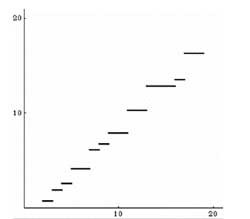It’s not quite the function $\pi(x)$ (counting the number of primes smaller than x) but it sure contains enough information to obtain this provided we have a way of describing $\Psi(x)$.

The Riemann zeta function (or rather $~(s-1)\zeta(s)$) has two product descriptions, the Hadamard product formula (running over all zeroes, both the trivial ones at $-2n$ and those in the critical strip), which is valid for all complex s and the Euler product valid for all $Re(s) > 1$. This will allow us to calculate in two different ways $\zeta'(s)/\zeta(s)$ which in turn allows us to have an explicit description of $\Psi(s)$ known as the Von Mangoldt formula

$\Psi(x) = x – \frac{1}{2}log(1 – \frac{1}{x^2}) – log(2 \pi) – \sum_{\rho} \frac{x^{\rho}}{\rho}$

where only the last term depends on the zeta-zeroes $\rho$ lying in the critical strip (and conjecturally all lying on the line $Re(x) = \frac{1}{2}$. The first few terms (those independent of the zeroes) give a continuous approximation of $\Psi(x)$ but how on earth can we get from that approxamation (on the left) to the step-like function itself (on the right)?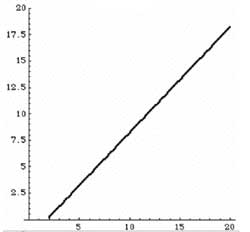We can group together zeta-zeroes $\rho=\beta + i \gamma$ with their comlex conjugate zeroes $\overline{\rho}$ and then one shows that the attribution to the Von Mangoldt formula is

$\frac{x^{\rho}}{\rho} + \frac{x^{\overline{\rho}}}{\overline{rho}} = \frac{2 x^{\beta}}{| \rho | }cos(\gamma log(x) – arctan(\gamma/\beta))$

Ignoring the term $x^{\beta}$ this is a peridodic function with amplitude $2/| \rho |$ (so getting smaller for larger and larger zeroes) and period $2\pi/ \gamma$. If the Riemann hypothesis holds (meaning that $\beta=1/2$ for all zeroes) one can even split a term in this contribution of every zero as a sort of ‘universal amplitude’. What is left is then a sum of purely periodic functions which a physicist will view as a superposition of (sound) waves and that is the music played by the primes!

Below, a video of the influence of adding the first 100 zeroes to a better and better approximation of $\Psi(x)$ (again taken from the Riemann page by Jeffrey Stopple). Surely watching the video will convince anyone of the importance of the Riemann zeta-zeroes to the prime-counting problem..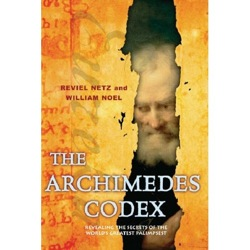Vacation is always a good time to catch up on some reading. Besides, there’s very little else you can do at night in a ski-resort… This year, I’ve taken along The Archimedes Codex: Revealing The Secrets Of The World’s Greatest Palimpsest by Reviel Netz and William Noel telling the story of the Archimedes Palimpsest.

The most remarkable of the above works is The Method, of which the palimpsest contains the only known copy. In his other works, Archimedes often proves the equality of two areas or volumes with his method of double contradiction: assuming that the first is bigger than the second leads to a contradiction, as does the assumption that the first be smaller than the second; so the two must be equal. These proofs, still considered to be rigorous and correct, used what we might now consider secondary-school geometry with rare brilliance. Later writers often criticized Archimedes for not explaining how he arrived at his results in the first place. This explanation is contained in The Method.
Essentially, the method consists in dividing the two areas or volumes in infinitely many stripes of infinitesimal width, and “weighing” the stripes of the first figure against those of the second, evaluated in terms of a finite Egyptian fraction series. He considered this method as a useful heuristic but always made sure to prove the results found in this manner using the rigorous arithmetic methods mentioned above.
He was able to solve problems that would now be treated by integral calculus, which was formally invented in the 17th century by Isaac Newton and Gottfried Leibniz, working independently. Among those problems were that of calculating the center of gravity of a solid hemisphere, the center of gravity of a frustum of a circular paraboloid, and the area of a region bounded by a parabola and one of its secant lines. Contrary to exaggerations found in some 20th century calculus textbooks, he did not use anything like Riemann sums, either in the work embodied in this palimpsest or in any of his other works. (For explicit details of the method used, see Archimedes’ use of infinitesimals.)
A problem solved exclusively in the Method is the calculation of the volume of a cylindrical wedge, a result that reappears as theorem XVII (schema XIX) of Kepler’s Stereometria.
Some pages of the Method remained unused by the author of the Palimpsest and thus they are still lost. Between them, an announced result concerned the volume of the intersection of two cylinders, a figure that Apostol and Mnatsakian have renamed n = 4 Archimedean globe (and the half of it, n = 4 Archimedean dome), whose volume relates to the n-polygonal pyramid.
In Heiberg’s time, much attention was paid to Archimedes’ brilliant use of infinitesimals to solve problems about areas, volumes, and centers of gravity. Less attention was given to the Stomachion, a problem treated in the Palimpsest that appears to deal with a children’s puzzle. Reviel Netz of Stanford University has argued that Archimedes discussed the number of ways to solve the puzzle. Modern combinatorics leads to the result that this number is 17,152. Due to the fragmentary state of the palimpsest it is unknown whether or not Archimedes came to the same result. This may have been the most sophisticated work in the field of combinatorics in Greek antiquity.

Also I hope to finish the novel Interred with their bones by Jennifer Lee Carrell (though I prefer the Dutch title, “Het Shakespeare Geheim” that is, “The Shakespeare Secret”) on a lost play by Shakespeare, and have a re-read of The music of the primes as I’ll use this book for my course starting next week.

(via the Arcadian Functor) At the time of the doing the Perelman-post someone rightfully commented that “making a voluntary retreat from the math circuit to preserve one’s own well-being (either mental, physical, scientific …)” should rather be called doing the Grothendieck as he was the first to pull this stunt.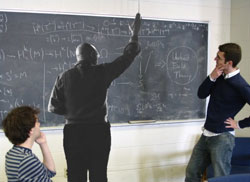On Facebook a couple of people have created the group The Petition for Alexander Grothendieck to Return from Exile. As you need to sign-up to Facebook to use this link and some of you may not be willing to do so, let me copy the description.

Alexander Grothendieck was born in Berlin, Germany on March 28, 1928. He was one of the most important and enigmatic mathematicians of the 20th century. After a lengthy and very productive career, highlighted by the awarding of the Fields Medal and the Crafoord Prize (the latter of which he declined), Grothendieck disappeared into the French countryside and ceased all mathematical activity. Grothendieck has lived in self-imposed exile since 1991.

We recently spotted Grothendieck in the “Gentleman’s Choice” bar in Montreal, Quebec. He was actually a really cool guy, and we spoke with him for quite some time. After a couple of rounds (on us) we were able to convince him to return from exile, under one stipulation – we created a facebook petition with 1729 mathematician members!

If 1729 mathematicians join this group, then Alexander Grothendieck will return from exile!!

1729 being of course the taxicab-curve number. The group posts convincing photographic evidence (see above) for their claim, has already 201 members (the last one being me) and has this breaking news-flash

Last week Grothendieck, or “the ‘Dieck” as we affectionately refer to him, returned to Montreal for a short visit to explain some of the theories he has been working on over the past decade. In particular, he explained how he has generalised the theory of schemes even further, to the extent that the Riemann Hypothesis and a Unified Field Theory are both trivial consequences of his work.

You know what to do!

By now, everyone remotely interested in Connes’ approach to the Riemann hypothesis, knows the _one line mantra_

one can use noncommutative geometry to extend Weil’s proof of the Riemann-hypothesis in the function field case to that of number fields

But, can one go beyond this sound-bite in a series of blog posts? A few days ago, I was rather optimistic, but now, after reading-up on the Connes-Consani-Marcolli project, I feel overwhelmed by the sheer volume of their work (and by my own ignorance of key tools in the approach). The most recent account takes up half of the 700+ pages of the book Noncommutative Geometry, Quantum Fields and Motives by Alain Connes and Matilde Marcolli…

So let us set a more modest goal and try to understand one of the first papers Alain Connes wrote about the RH : Noncommutative geometry and the Riemann zeta function. It is only 24 pages long and relatively readable. But even then, the reader needs to know about class field theory, the classification of AF-algebras, Hecke algebras, etc. etc. Most of these theories take a book to explain. For example, the first result he mentions is the main result of local class field theory which appears only towards the end of the 200+ pages of Jean-Pierre Serre’s Local Fields, itself a somewhat harder read than the average blogpost…

Anyway, we will see how far we can get. Here’s the plan : I’ll take the heart-bit of their approach : the Bost-Connes system, and will try to understand it from an algebraist’s viewpoint. Today we will introduce the groups involved and describe their cosets.

For any commutative ring $R$ let us consider the group of triangular $2 \times 2$ matrices of the form

$P_R = { \begin{bmatrix} 1 & b \\ 0 & a \end{bmatrix}~|~b \in R, a \in R^* }$

(that is, $a$ in an invertible element in the ring $R$). This is really an affine group scheme defined over the integers, that is, the coordinate ring

$\mathbb{Z}[P] = \mathbb{Z}[x,x^{-1},y]$ becomes a Hopf algebra with comultiplication encoding the group-multiplication. Because

$\begin{bmatrix} 1 & b_1 \\ 0 & a_1 \end{bmatrix} \begin{bmatrix} 1 & b_2 \\ 0 & a_2 \end{bmatrix} = \begin{bmatrix} 1 & 1 \times b_2 + b_1 \times a_2 \\ 0 & a_1 \times a_2 \end{bmatrix}$

we have $\Delta(x) = x \otimes x$ and $\Delta(y) = 1 \otimes y + y \otimes x$, or $x$ is a group-like element whereas $y$ is a skew-primitive. If $R \subset \mathbb{R}$ is a subring of the real numbers, we denote by $P_R^+$ the subgroup of $P_R$ consisting of all matrices with $a > 0$. For example,

$\Gamma_0 = P_{\mathbb{Z}}^+ = { \begin{bmatrix} 1 & n \\ 0 & 1 \end{bmatrix}~|~n \in \mathbb{Z} }$

which is a subgroup of $\Gamma = P_{\mathbb{Q}}^+$ and our first job is to describe the cosets.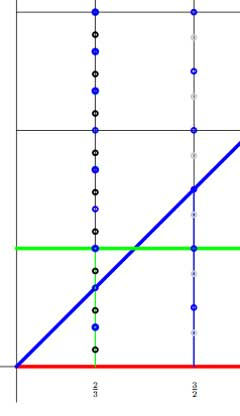The left cosets $\Gamma / \Gamma_0$ are the subsets $\gamma \Gamma_0$ with $\gamma \in \Gamma$. But,

$\begin{bmatrix} 1 & b \\ 0 & a \end{bmatrix} \begin{bmatrix} 1 & n \\ 0 & 1 \end{bmatrix} = \begin{bmatrix} 1 & b+n \\ 0 & a \end{bmatrix}$

so if we represent the matrix $\gamma = \begin{bmatrix} 1 & b \\ 0 & a \end{bmatrix}$ by the point $~(a,b)$ in the right halfplane, then for a given positive rational number $a$ the different cosets are represented by all $b \in [0,1) \cap \mathbb{Q} = \mathbb{Q}/\mathbb{Z}$. Hence, the left cosets are all the rational points in the region between the red and green horizontal lines. For fixed $a$ the cosets correspond to the rational points in the green interval (such as over $\frac{2}{3}$ in the picture on the left.

Similarly, the right cosets $\Gamma_0 \backslash \Gamma$ are the subsets $\Gamma_0 \gamma$ and as

$\begin{bmatrix} 1 & n \\ 0 & 1 \end{bmatrix} \begin{bmatrix} 1 & b \\ 0 & a \end{bmatrix} = \begin{bmatrix} 1 & b+na \\ 0 & a \end{bmatrix}$

we see similarly that the different cosets are precisely the rational points in the region between the lower red horizontal and the blue diagonal line. So, for fixed $a$ they correspond to rational points in the blue interval (such as over $\frac{3}{2}$) $[0,a) \cap \mathbb{Q}$. But now, let us look at the double coset space $\Gamma_0 \backslash \Gamma / \Gamma_0$. That is, we want to study the orbits of the action of $\Gamma_0$, acting on the right, on the left-cosets $\Gamma / \Gamma_0$, or equivalently, of the action of $\Gamma_0$ acting on the left on the right-cosets $\Gamma_0 \backslash \Gamma$. The crucial observation to make is that these actions have finite orbits, or equivalently, that $\Gamma_0$ is an almost normal subgroup of $\Gamma$ meaning that $\Gamma_0 \cap \gamma \Gamma_0 \gamma^{-1}$ has finite index in $\Gamma_0$ for all $\gamma \in \Gamma$. This follows from

$\begin{bmatrix} 1 & n \\ 0 & 1 \end{bmatrix} \begin{bmatrix} 1 & b \\ 0 & a \end{bmatrix} \begin{bmatrix} 1 & m \\ 0 & 1 \end{bmatrix} = \begin{bmatrix} 1 & b+m+an \\ 0 & a \end{bmatrix}$

and if $n$ varies then $an$ takes only finitely many values modulo $\mathbb{Z}$ and their number depends only on the denominator of $a$. In the picture above, the blue dots lying on the line over $\frac{2}{3}$ represent the double coset

$\Gamma_0 \begin{bmatrix} 1 & \frac{2}{3} \\ 0 & \frac{2}{3} \end{bmatrix}$ and we see that these dots split the left-cosets with fixed value $a=\frac{2}{3}$ (that is, the green line-segment) into three chunks (3 being the denominator of a) and split the right-cosets (the line-segment under the blue diagonal) into two subsegments (2 being the numerator of a). Similarly, the blue dots on the line over $\frac{3}{2}$ divide the left-cosets in two parts and the right cosets into three parts.

This shows that the $\Gamma_0$-orbits of the right action on the left cosets $\Gamma/\Gamma_0$ for each matrix $\gamma \in \Gamma$ with $a=\frac{2}{3}$ consist of exactly three points, and we denote this by writing $L(\gamma) = 3$. Similarly, all $\Gamma_0$-orbits of the left action on the right cosets $\Gamma_0 \backslash \Gamma$ with this value of a consist of two points, and we write this as $R(\gamma) = 2$.

For example, on the above picture, the black dots on the line over $\frac{2}{3}$ give the matrices in the double coset of the matrix

$\gamma = \begin{bmatrix} 1 & \frac{1}{7} \\ 0 & \frac{2}{3} \end{bmatrix}$

and the gray dots on the line over $\frac{3}{2}$ determine the elements of the double coset of

$\gamma^{-1} = \begin{bmatrix} 1 & -\frac{3}{14} \\ 0 & \frac{3}{2} \end{bmatrix}$

and one notices (in general) that $L(\gamma) = R(\gamma^{-1})$. But then, the double cosets with $a=\frac{2}{3}$ are represented by the rational b’s in the interval $[0,\frac{1}{3})$ and those with $a=\frac{3}{2}$ by the rational b’s in the interval $\frac{1}{2}$. In general, the double cosets of matrices with fixed $a = \frac{r}{s}$ with $~(r,s)=1$ are the rational points in the line-segment over $a$ with $b \in [0,\frac{1}{s})$.

That is, the Bost-Connes double coset space $\Gamma_0 \backslash \Gamma / \Gamma_0$ are the rational points in a horrible fractal comb. Below we have drawn only the part of the dyadic values, that is when $a = \frac{r}{2^t}$ in the unit inverval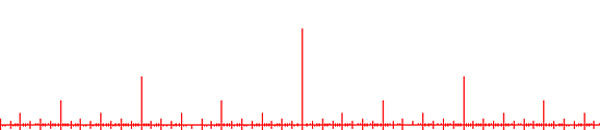and of course we have to super-impose on it similar pictures for rationals with other powers as their denominators. Fortunately, NCG excels in describing such fractal beasts…

UPDATE : here is a slightly beter picture of the coset space, drawing the part over all rational numbers contained in the 15-th Farey sequence. The blue segments of length one are at 1,2,3,…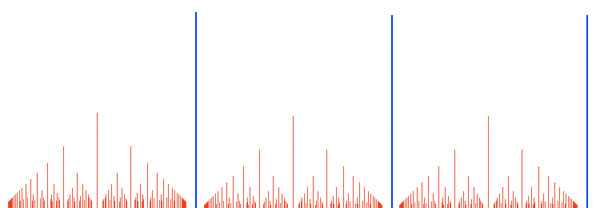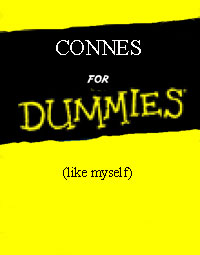You may not have noticed, but the really hard work was done behind the scenes, resurrecting about 300 old posts (some of them hidden by giving them ‘private’-status). Ive only deleted about 10 posts with little or no content and am sorry I’ve self-destructed about 20-30 hectic posts over the years by pressing the ‘delete post’ button. I would have liked to reread them after all the angry mails Ive received. But, as Ive defended myself at the time, and as I continue to do today, a blog only records feelings at a specific moment. Often, the issue is closed for me once Ive put my frustrations in a post, and then Ill forget all about it. Sadly, the gossip-circuit in noncommutative circles is a lot, a lot, slower than my mood swings, so by the time people complain it’s no longer an issue for me and I tend to delete the post altogether. A blog really is a sort of diary. For example, it only struck me now, rereading the posts of the end of 2006, beginning of 2007, how depressed I must have been at the time. Fortunately, life has improved, somewhat… Still, after all these reminiscences, the real issue is : what comes next?

Some of you may have noticed that I’ve closed the open series on tori-cryptography and on superpotentials in a rather abrupt manner. It took me that long to realize that none of you is waiting for this kind of posts. You’re thinking : if he really wants to show off, let him do his damned thing on the arXiv, a couple of days a year, at worst, and then we can then safely ignore it, like we do with most papers. Isnt’t that true? Of course it is…

So, what are you waiting for? Here’s what I believe to be a sensible thing to try out. Over the last 4 years I must have posted well over 50 times what I believe noncommutative geometry is all about, so if you still don’t know, please consult the archive, I fear I can only repeat myself. Probably, it is more worthwhile to reach out to other approaches to noncommutative geometry, trying to figure out what, if anything, they are after, without becoming a new-age convert (‘connes-vert’, I’d say). The top-left picture may give you an inkling of what I’m after… Besides, Im supposed to run a ‘capita selecta’ course for third year Bachelors and Ive chosen to read with them the book The music of the primes and to expand on the mathematics hinted only at in the book. So, I’ll totally immerse myself in Connes’ project to solve the Riemann-hypothesis in the upcoming months.

Again, rereading old posts, it strikes me how much effort I’ve put into trying to check whether technology can genuinely help mathematicians to do what they want to do more efficiently (all post categorized as iMath). I plan some series of posts re-exploring these ideas. The first series will be about the overhyped Web-2 thing of social-bookmarking. So, in the next weeks I’ll go undercover and check out which socialsites are best for mathematicians (in particular, noncommutative geometers) to embrace…

Apart from these, admittedly vague, plans I am as always open for suggestions you might have. So, please drop a comment..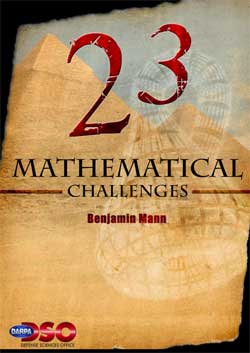Via the n-category cafe (and just now also the Arcadian functor ) I learned that Benjamin Mann of DARPA has constructed a list of 23 challenges for mathematics for this century.

DARPA is the “Defense Advanced Research Projects Agency” and is an agency of the United States Department of Defense ‘responsible for the development of new technology for use by the military’.

Bejamin Mann is someone in their subdivision DSO, that is, the “Defense Sciences Office” that ‘vigorously pursues the most promising technologies within a broad spectrum of the science and engineering research communities and develops those technologies into important, radically new military capabilities’.

I’m not the greatest fan of the US military, but the proposed list of 23 mathematical challenges is actually quite original and interesting.

What follows is my personal selection of what I consider the top 5 challenges from the list (please disagree) :

1. The Mathematics of Quantum Computing, Algorithms, and Entanglement (DARPA 15) : “In the last century we learned how quantum phenomena shape
our world. In the coming century we need to develop the
mathematics required to control the quantum world.”

2. Settle the Riemann Hypothesis (DARPA 19) : “The Holy Grail of number theory.”

3. Geometric Langlands and Quantum Physics (DARPA 17) : “How does the Langlands program, which originated in number
theory and representation theory, explain the fundamental
symmetries of physics? And vice versa?”

4. The Geometry of Genome Space (DARPA 15) : “What notion of distance is needed to incorporate biological utility?”

5. Algorithmic Origami and Biology (DARPA 10) : “Build a stronger mathematical theory for isometric and rigid
embedding that can give insight into protein folding.”

All of this will have to wait a bit, for now

HAPPY & HEALTHY 2008

In a couple of days I’ll be blogging for 4 years… and I’m in the process of resurrecting about 300 posts from a database-dump made in june. For example here’s my first post ever which is rather naive. This conversion program may last for a couple of weeks and I apologize for all unwanted pingbacks it will produce.

I’ll try to convert chunks of related posts in one go, so that I can at least give them correct self-references. Today’s work consisted in rewriting the posts of my virtual course, in march of this year, on dessins d’enfants and its connection to noncommutative geometry (a precursor of what Ive been blogging about recently). These posts were available through the PDF-archive but are from now on open to the internal search-function. Here are the internal links and a short description of their contents

Besides, I’ve added a few scattered old posts, many more to follow…

Yesterday, Jan Stienstra gave a talk at theARTS entitled “Quivers, superpotentials and Dimer Models”. He started off by telling that the talk was based on a paper he put on the arXiv Hypergeometric Systems in two Variables, Quivers, Dimers and Dessins d’Enfants but that he was not going to say a thing about dessins but would rather focuss on the connection with superpotentials instead…pleasing some members of the public, while driving others to utter despair.

Anyway, it gave me the opportunity to figure out for myself what dessins might have to do with dimers, whathever these beasts are. Soon enough he put on a slide containing the definition of a dimer and from that moment on I was lost in my own thoughts… realizing that a dessin d’enfant had to be a dimer for the Dedekind tessellation of its associated Riemann surface!
and a few minutes later I could slap myself on the head for not having thought of this before :

There is a natural way to associate to a Farey symbol (aka a permutation representation of the modular group) a quiver and a superpotential (aka a necklace) defining (conjecturally) a Calabi-Yau algebra! Moreover, different embeddings of the cuboid tree diagrams in the hyperbolic plane may (again conjecturally) give rise to all sorts of arty-farty fanshi-wanshi dualities…

I’ll give here the details of the simplest example I worked out during the talk and will come back to general procedure later, when I’ve done a reference check. I don’t claim any originality here and probably all of this is contained in Stienstra’s paper or in some physics-paper, so if you know of a reference, please leave a comment. Okay, remember the Dedekind tessellation ?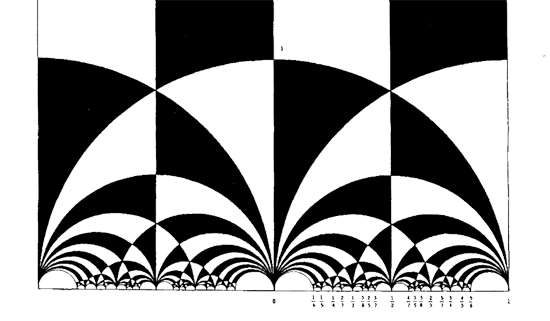So, all hyperbolic triangles we will encounter below are colored black or white. Now, take a Farey symbol and consider its associated special polygon in the hyperbolic plane. If we start with the Farey symbol

$$\xymatrix{\infty \ar@{-}_{(1)}[r] & 0 \ar@{-}_{\bullet}[r] & 1 \ar@{-}_{(1)}[r] & \infty}$$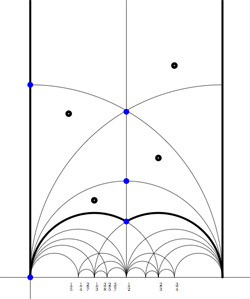we get the special polygonal region bounded by the thick edges, the vertical edges are identified as are the two bottom edges. Hence, this fundamental domain has 6 vertices (the 5 blue dots and the point at $i \infty$) and 8 hyperbolic triangles (4 colored black, indicated by a black dot, and 4 white ones).

Right, now let us associate a quiver to this triangulation (which embeds the quiver in the corresponding Riemann surface). The vertices of the triangulation are also the vertices of the quiver (so in our case we are going for a quiver with 6 vertices). Every hyperbolic edge in the triangulation gives one arrow in the quiver between the corresponding vertices. The orientation of the arrow is determined by the color of a triangle of which it is an edge : if the triangle is black, we run around its edges counter-clockwise and if the triangle is white we run over its edges clockwise (that is, the orientation of the arrow is independent of the choice of triangles to determine it). In our example, there is one arrows directed from the vertex at $i$ to the vertex at $0$, whether you use the black triangle on the left to determine the orientation or the white triangle on the right. If we do this for all edges in the triangulation we arrive at the quiver below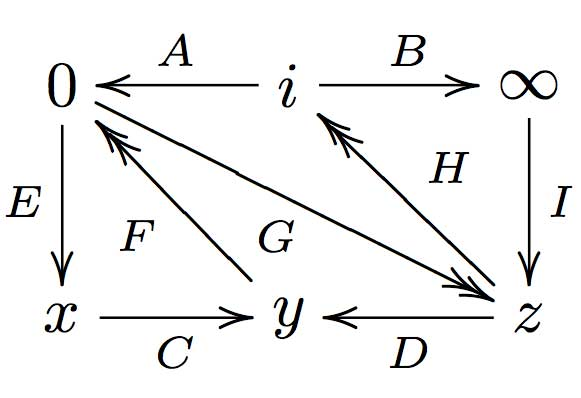where x,y and z are the three finite vertices on the $\frac{1}{2}$-axis from bottom to top and where I’ve used the physics-convention for double arrows, that is there are two F-arrows, two G-arrows and two H-arrows. Observe that the quiver is of Calabi-Yau type meaning that there are as much arrows coming into a vertex as there are arrows leaving the vertex.

Now that we have our quiver we determine the superpotential as follows. Fix an orientation on the Riemann surface (for example counter-clockwise) and sum over all black triangles the product of the edge-arrows counterclockwise MINUS sum over all white triangles
the product of the edge arrows counterclockwise. So, in our example we have the cubic superpotential

$IH’B+HAG+G’DF+FEC-BHI-H’G’A-GFD-CEF’$

From this we get the associated noncommutative algebra, which is the quotient of the path algebra of the above quiver modulo the following ‘commutativity relations’

$\begin{cases} GH &=G’H’ \\ IH’ &= IH \\ FE &= F’E \\ F’G’ &= FG \\ CF &= CF’ \\ EC &= GD \\ G’D &= EC \\ HA &= DF \\ DF’ &= H’A \\ AG &= BI \\ BI &= AG’ \end{cases}$

and morally this should be a Calabi-Yau algebra (( can someone who knows more about CYs verify this? )). This concludes the walk through of the procedure. Summarizing : to every Farey-symbol one associates a Calabi-Yau quiver and superpotential, possibly giving a Calabi-Yau algebra!

Here the details of the iguanodon series. Start with the Farey sequence $F(n)$of order n which is the sequence of completely reduced fractions between 0 and 1 which, when in lowest terms, have denominators less than or equal to n, arranged in order of increasing size. Here are the first eight Fareys

F(1) = {0⁄1, 1⁄1}
F(2) = {0⁄1, 1⁄2, 1⁄1}
F(3) = {0⁄1, 1⁄3, 1⁄2, 2⁄3, 1⁄1}
F(4) = {0⁄1, 1⁄4, 1⁄3, 1⁄2, 2⁄3, 3⁄4, 1⁄1}
F(5) = {0⁄1, 1⁄5, 1⁄4, 1⁄3, 2⁄5, 1⁄2, 3⁄5, 2⁄3, 3⁄4, 4⁄5, 1⁄1}
F(6) = {0⁄1, 1⁄6, 1⁄5, 1⁄4, 1⁄3, 2⁄5, 1⁄2, 3⁄5, 2⁄3, 3⁄4, 4⁄5, 5⁄6, 1⁄1}
F(7) = {0⁄1, 1⁄7, 1⁄6, 1⁄5, 1⁄4, 2⁄7, 1⁄3, 2⁄5, 3⁄7, 1⁄2, 4⁄7, 3⁄5, 2⁄3, 5⁄7, 3⁄4, 4⁄5, 5⁄6, 6⁄7, 1⁄1}
F(8) = {0⁄1, 1⁄8, 1⁄7, 1⁄6, 1⁄5, 1⁄4, 2⁄7, 1⁄3, 3⁄8, 2⁄5, 3⁄7, 1⁄2, 4⁄7, 3⁄5, 5⁄8, 2⁄3, 5⁄7, 3⁄4, 4⁄5, 5⁄6, 6⁄7, 7⁄8, 1⁄1}Farey sequences have plenty of mysterious properties. For example, in 1924 J. Franel and Edmund Landau proved that an asymptotic density result about Farey sequences is equivalent to the Riemann hypothesis.
More precisely, let a(n) be the number of terms in the Farey sequence F(n) (that is, a(1)=2,a(2)=3,…,a(8)=23 etc. This is sequence A005728 in the online integer sequences catalog).
Let $F(n)_j$ denote the j-th term in F(n), then the following conjecture is equivalent to the Riemann hypothesis

For every $\epsilon > 0$ there is a constant C depending on $\epsilon$ such that

$\sum_{j=1}^{a(n)} | F(n)_j – \frac{j}{a(n)} | < C n^{\frac{1}{2}+\epsilon}$

when n goes to infinity. Anyway, let us continue our construction. Farey sequences are clearly symmetric around 1/2 so let us just take half of them, so we jump to 1 when we have reached 1/2. Let us extend this halved Farey on both sides with $\infty$ and call it the modified Farey sequence f(n). For example,

$f(3) = {~\infty,0,\frac{1}{3},\frac{1}{2},1,\infty }$

Now consider the Farey code in which we identify the two sides connected to $\infty$ and mark two consecutive Farey numbers as

$$\xymatrix{f(n)_i \ar@{-}[r]_{\bullet} & f(n)_{i+1}}$$

That is, the Farey code associated to the modified sequence f(3) is

$$\xymatrix{\infty \ar@{-}[r]_{1} & 0 \ar@{-}[r]_{\bullet} & \frac{1}{3} \ar@{-}[r]_{\bullet} & \frac{1}{2} \ar@{-}[r]_{\bullet} & 1 \ar@{-}[r]_{1} & \infty}$$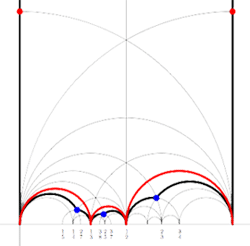Recall from earlier that to a Farey-code we can associate a special polygon by first taking the hyperbolic convex hull of all the terms in the sequence (the region bounded by the vertical lines and the bottom red circles in the picture on the left) and adding to it for each odd interval $$\xymatrix{f(n)_i \ar@{-}[r]_{\bullet} & f(n)_{i+1}}$$ the triangle just outside the convex hull consisting of two odd edges in the Dedekind tessellation (then we obtain the region bounded by the black geodesics for the sequence f(3)).

Next, we can associate to this special polygon a cuboid tree diagram by considering all even and odd vertices on the boundary (which are tinted red, respectively blue) together with all odd vertices in the interior of the special polygon. These are indicated in the left picture below. If we connect these vertices with the geodesics in the polygon we get a cuboid tree diagram. The obtained cuboid tree diagram is depicted on the right below.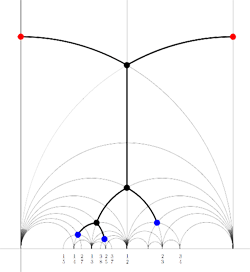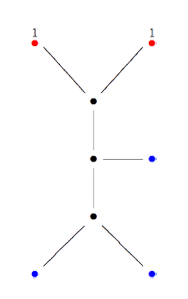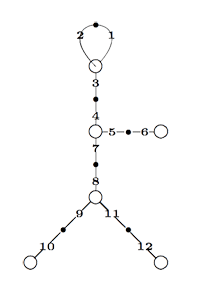Finally, identifying the red points (as they lie on geodesics connected to $\infty$ which are identified in the Farey code), adding even points on the remaining geodesics and numbering the obtained half-lines we obtain the dessin d’enfant given on the left hand side. To such a dessin we can associate its monodromy group which is a permutation group on the half-lines generated by an order two element indicating which half-lines make up a line and an order three element indicating which half-lines one encounters by walking counter-clockwise around a three-valent vertex. For the dessin on the left the group is therefore the subgroup of $S_{12}$ generated by the elements

$\alpha = (1,2)(3,4)(5,6)(7,8)(9,10)(11,12)$

$\beta = (1,2,3)(4,5,7)(8,9,11)$

and a verification with GAP tells us that this group is the sporadic Mathieu group $M_{12}$. This concludes the description of the second member of the Iguanodon series. If you like to check that the first 8 iguanodons are indeed the simple groups

$L_2(7), M_{12}, A_{16}, M_{24}, A_{28}, A_{40}, A_{48}, A_{60}, \ldots$

the following dissection of the Iguanodon may prove useful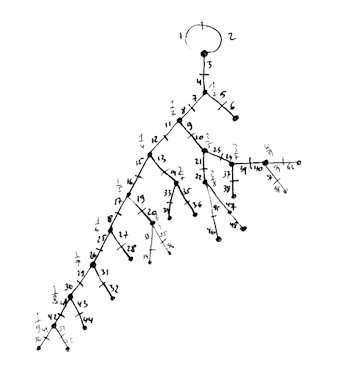Im in the process of writing/revising/extending the course notes for next year and will therefore pack more math-books than normal.

These are for a 3rd year Bachelor course on Algebraic Geometry and a 1st year Master course on Algebraic and Differential Geometry. The bachelor course was based this year partly on Miles Reid’s Undergraduate Algebraic Geometry and partly on David Mumford’s Red Book, but this turned out to be too heavy going. Next year I’ll be happy if they know enough on algebraic curves. The backbone of these two courses will be Fulton’s old but excellent Algebraic curves. It’s self contained (unlike Hartshorne’s book that assumes a prior course on commutative algebra), contains a lot of exercises and goes on to the Brill-Noether proof of Riemann-Roch. Still, Id like to extend it with the introductory chapter and the chapters on Riemann surfaces from Complex Algebraic Curves by Frances Kirwan, a bit on elliptic and modular functions from Elliptic curves by Henry McKean and Victor Moll and the adelic proof of Riemann-Roch and applications of it to the construction of algebraic codes from Algebraic curves over finite fields by Carlos Moreno. If time allows Id love to include also the chapter on zeta functions but I fear this will be difficult.

These are to spice up a 2nd year Bachelor course on Representations of Finite Groups with a tiny bit of Galois representations, both as motivation and to wet their appetite for elliptic curves and algebraic geometry. Ive received Fearless Symmetry by Avner Ash and Robert Gross only yesterday and find it hard to stop reading. It attempts to explain Galois representations and generalized reciprocity laws to a general audience and from what I read so far, they really do a terrific job. Another excellent elementary introduction to elliptic curves and Galois representations is in Invitation to the Mathematics of Fermat-Wiles by Yves Hellegouarch. On a gossipy note, the appendix “The origin of the elliptic approach to Fermat’s last theorem” is fun reading. Finally, Ill also take Introduction to Fermat’s Last Theorem by Alf van der Poorten along simply because I love his writing style.

These are included just for fun. The Poincare Conjecture by Donal O’Shea because I know far too little about it, Letters to a Young Mathematician by Ian Stewart because I like the concept of the book and finally The sensual (quadratic) form by John Conway because I need to have at all times at least one Conway-book nearby.